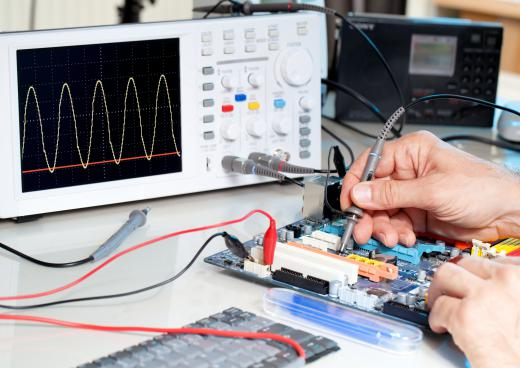# What Is a Potential Difference?

Joe Williams
Joe Williams

A potential difference is the energy per unit charge in an electrical circuit. Potential difference must be measured between two points because it is generally impractical to measure the electrical potential of a single point. The potential difference of a point in an electrical circuit is typically made with respect to a reference potential, such as the common ground.

Potential difference is commonly known as voltage because volts are the standard unit of measure for this quantity. It is also known historically as pressure and tension. "Pressure" is an obsolete term for potential difference, but it is still used to refer to potential difference within the context of vacuum tube electronics.

Voltage also can be viewed as the energy needed to move a charge between points divided by the magnitude of that charge. This energy total must include both the static and dynamic energy required to move the charge. The calculation of potential difference becomes more accurate as the magnitude of the measured charge becomes smaller.An oscilloscope can be used to measure potential difference.

A quantity of potential difference must include a sign depending on the direction in which the electrons are flowing. The definition of potential difference assumes that positively charged particles move toward lower voltages and negatively charged particles move toward higher voltages. This means that the electrons in a circuit flow from a point of higher voltage to a point of lower voltage.

The description of potential difference frequently uses water as an analogy for electricity. This analogy assumes that a mechanical pump is driving water through a closed circuit of pipes. The potential difference in this analogy is the difference in water pressure between two points in the circuit. Water therefore will flow between the two points if there is a difference in their pressure, allowing the water to perform work. Similarly, an electrical circuit can perform work only if a potential difference exists between two points in the circuit.

Various instruments measure potential difference depending on their method of operation. A voltmeter has two leads, each of which connects to a point in the circuit. This device measures the drop in voltage across a fixed resistor, which is proportional to amount of volts in the circuit.

A potentiometer compares an unknown voltage to a known voltage in the circuit. An oscilloscope amplifies the voltage in the circuit, which deflects an electron beam. The amount of deflection is proportional to the voltage in the circuit. A multimeter measures several electrical quantities, including voltage.